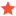Rotate the cameramibeJunior MemberPosts: 2 Threads: 1 Joined: Jan 2014 Reputation: 0 01-16-2014, 03:50 PM Hi, I´ve successfully realized the Rotation of a camera by code: Code:```var rotateTransform3D = new RotateTransform3D { CenterX = 0, CenterZ = 0 };             var axisAngleRotation3D = new AxisAngleRotation3D { Axis = new Vector3D(0, 1, 0), Angle = Convert.ToDouble(Slider2.Value) };             rotateTransform3D.Rotation = axisAngleRotation3D;             _robotArmReader3ds.Cameras.Transform = rotateTransform3D;``` I´m using a slider to set the rotation angle. I want to rotate all 3 axes of the camera: Therefore I implemented 3 slides with the following codes: Rotating x-axis: Code:```var rotateTransform3D = new RotateTransform3D { CenterX = 0, CenterZ = 0 };             var axisAngleRotation3D = new AxisAngleRotation3D { Axis = new Vector3D(1, 0, 0), Angle = Convert.ToDouble(Slider1.Value) };             rotateTransform3D.Rotation = axisAngleRotation3D;             _robotArmReader3ds.Cameras.Transform = rotateTransform3D;``` Rotating y-axis: Code:```var rotateTransform3D = new RotateTransform3D { CenterX = 0, CenterZ = 0 };             var axisAngleRotation3D = new AxisAngleRotation3D { Axis = new Vector3D(0, 1, 0), Angle = Convert.ToDouble(Slider2.Value) };             rotateTransform3D.Rotation = axisAngleRotation3D;             _robotArmReader3ds.Cameras.Transform = rotateTransform3D;``` And rotating z-Axis: Code:```var rotateTransform3D = new RotateTransform3D { CenterX = 0, CenterZ = 0 };             var axisAngleRotation3D = new AxisAngleRotation3D { Axis = new Vector3D(0, 0, 1), Angle = Convert.ToDouble(Slider3.Value) };             rotateTransform3D.Rotation = axisAngleRotation3D;             _robotArmReader3ds.Cameras.Transform = rotateTransform3D;``` The problem is, that I rotate the x-axis by 30°. Than I rotate the y-axis by 20°. Now, the rotation of the x-axis is resetted to 0° first and the the y-Axis. How can I rotate all 3axis by 3 sliders, without resetting axis? michael Find ReplyabenedikAdministratorPosts: 662 Threads: 8 Joined: Sep 2009 Reputation: 10 01-22-2014, 03:21 PM I do not understand why you are not using any camera from Ab3d.PowerToys - here all the cameras have Heading, Attitude and Bank properties. You can bind sliders directly to the properties. Anyway, one of the problems with your code is that you have only one rotation assigned to Camera object - either is this x, y or z rotation - but always only one. This could be fixed by creating a TransformGroup and adding all transformations to that group. But this would not solve your problem - rotating is 3D is difficult: if you first rotate by 90 degress on x axis and than by 30 degrees on y axis you will get different result that if you first rotate by 30 degrees on y and than by 90 degrees on x axis. The math I use is quite complex and I do not want to disclose it here - just use the cameras from Ab3d.PowerToys and you will have the solution. Andrej Benedik Find ReplymibeJunior MemberPosts: 2 Threads: 1 Joined: Jan 2014 Reputation: 0 02-06-2014, 11:47 AM Hi, thank´s for your reply. I just checked the PowerToys. It seems to be much easier to use, instead of my first try with using the RotateTransform3D. :-) michael Find Reply

Forum Jump:

 Users browsing this thread: 1 Guest(s)